### Home > CALC > Chapter Ch3 > Lesson 3.2.2 > Problem3-63

3-63.
1.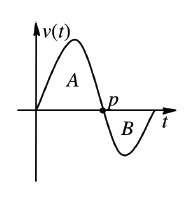Answer the following questions using the graph at right, which shows the velocity of a runner over time. The letters A and B represent the areas of the two regions in the diagram. Homework Help ✎

1. Describe the motion of the runner during the time that is illustrated in the graph.

2. What is the significance of point p

3. What does the area A represent in this situation? That is, what does it tell you about the runner? What about area B?

4. If A = 30 meters and B = −5 meters, what does A + B represent in this situation? Why is B negative?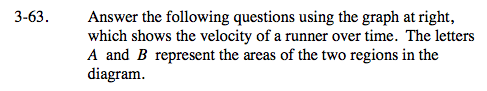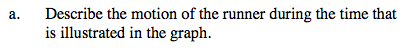Include information about the direction that the runner was moving.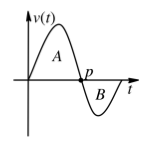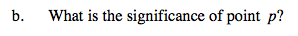How does the runner's motion change when s/he reaches point p?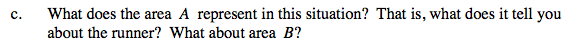What does area under a velocity curve represent?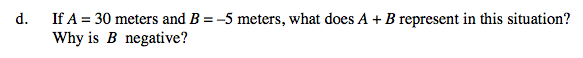Compare and contrast 'displacement' with 'total distance.'